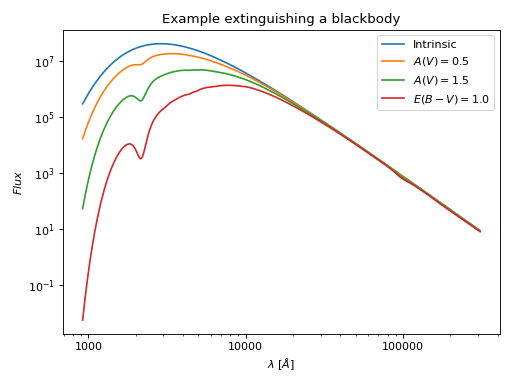# Extinguish or Unextinguish Data¶

Two of the three flavors of models include a function to calculate the factor to multiple (extinguish) or divide (unextinguish) a spectrum by to add or remove the effects of dust, respectively.

Extinguish is also often called reddening. Extinguishing a spectrum often reddens the flux, but sometimes ‘bluens’ the flux (e.g, on the short wavelength side of the 2175 A bump). So extinguish is the more generic term.

## Extinguish a Blackbody¶

import matplotlib.pyplot as plt
import numpy as np

import astropy.units as u
from astropy.modeling.models import BlackBody

from dust_extinction.parameter_averages import F19

# generate wavelengths between 0.12 and 3 microns
#    within the valid range for the F19 R(V) dependent relationship
lam = np.logspace(np.log10(0.12), np.log10(3.0), num=1000)

# setup the inputs for the blackbody function
wavelengths = lam*1e4*u.AA
temperature = 10000*u.K

# get the blackbody flux
bb_lam = BlackBody(10000*u.K, scale=1.0 * u.erg / (u.cm ** 2 * u.AA * u.s * u.sr))
flux = bb_lam(wavelengths)

# initialize the model
ext = F19(Rv=3.1)

# get the extinguished blackbody flux for different amounts of dust
flux_ext_av05 = flux*ext.extinguish(wavelengths, Av=0.5)
flux_ext_av15 = flux*ext.extinguish(wavelengths, Av=1.5)
flux_ext_ebv10 = flux*ext.extinguish(wavelengths, Ebv=1.0)

# plot the intrinsic and extinguished fluxes
fig, ax = plt.subplots()

ax.plot(wavelengths, flux, label='Intrinsic')
ax.plot(wavelengths, flux_ext_av05, label='$A(V) = 0.5$')
ax.plot(wavelengths, flux_ext_av15, label='$A(V) = 1.5$')
ax.plot(wavelengths, flux_ext_ebv10, label='$E(B-V) = 1.0$')

ax.set_xlabel('$\lambda$ [$\AA$]')
ax.set_ylabel('$Flux$')

ax.set_xscale('log')
ax.set_yscale('log')

ax.set_title('Example extinguishing a blackbody')

ax.legend(loc='best')
plt.tight_layout()
plt.show()## Notebooks¶

A great way to show how to use the dust_extinction package is using a jupyter notebook. Check out the Analyzing interstellar reddening and calculating synthetic photometry notebook that was created as part of the Learn.Astropy effort.